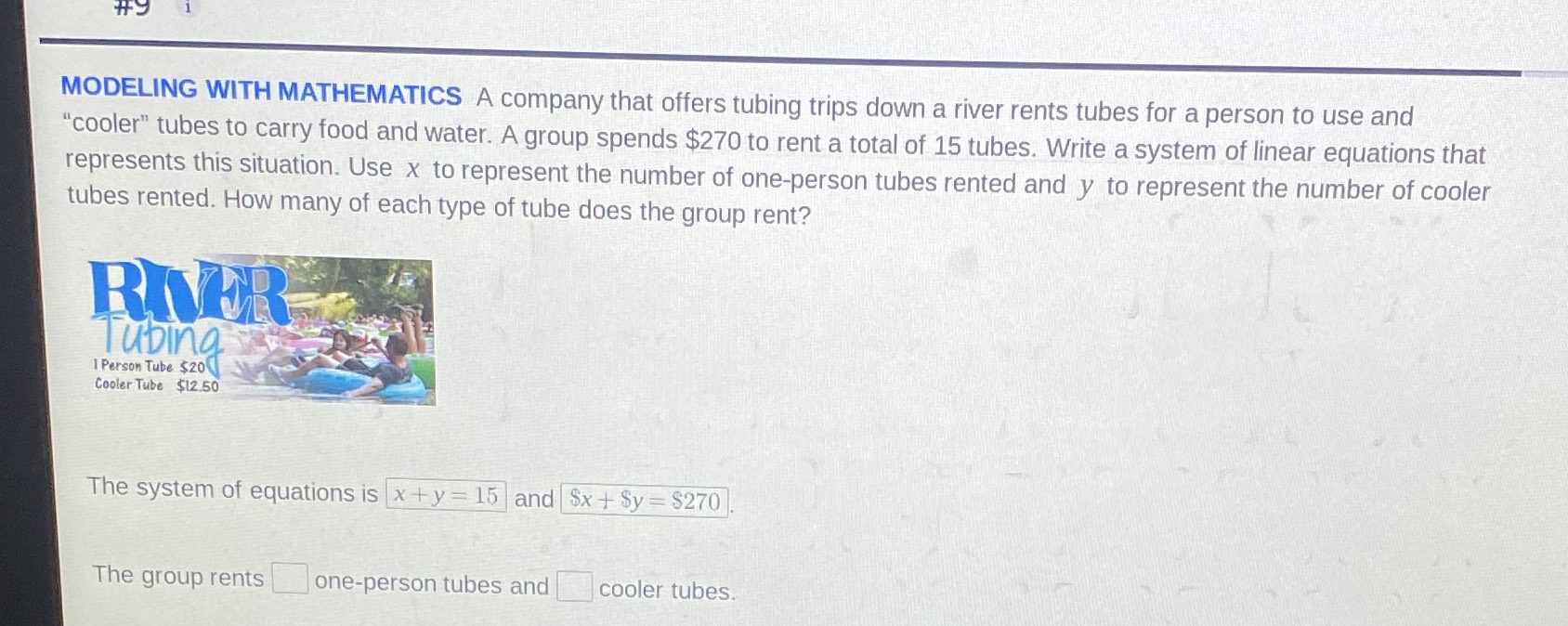### ¿Todavía tienes preguntas de matemáticas?

Pregunte a nuestros tutores expertos
Algebra
PreguntaA company that offers tubing trips down a river rents tubes for a person to use and "cooler" tubes to carry food and water. A group spends $$\ 270$$ to rent a total of 15 tubes. Write a system of linear equations that represents this situation. Use $$x$$ to represent the number of one-person tubes rented and $$y$$ to represent the number of cooler tubes rented. How many of each type of tube does the group rent?